# Simulating a Polarimetric Radar Return for Weather Observation

This example shows how to simulate a polarimetric Doppler radar return that meets the requirements of weather observations. Radar plays a critical role in weather observation, detection of hazards, classification and quantification of precipitation, and forecasting. In addition, polarimetric radar provides multiparameter measurements with unprecedented quality and information. This example shows how to simulate a polarimetric Doppler radar that scans an area of distributed weather targets. The simulation derives the radar parameters according to the well-known NEXRAD radar specifications. After synthesizing the received pulses, radar spectral moment estimation and polarimetric moment estimation are performed. The estimates are compared with NEXRAD ground truth, from which error statistics are obtained and data quality is evaluated.

Radar system specifications are designed as follows.

max_range = 100e3; % Maximum unambiguous range (m) range_res = 250; % Required range resolution (m) pulnum = 32; % Number of pulses to process in an azimuth fc = 2800e6; % Frequency (Hz) prop_speed = physconst('LightSpeed'); % Propagation speed (m/s) lambda = prop_speed/fc; % Wavelength (m) 

load NEXRAD_Parameters.mat 

### Antenna Pattern

As NEXRAD is polarimetric, modeling the polarimetric characteristics of the antenna and weather targets is important. According to NEXRAD specifications, the antenna pattern has a beamwidth of about 1 degree and first sidelobe below -30 dB.

azang = [-180:0.5:180]; elang = [-90:0.5:90]; % We synthesize a pattern using isotropic antenna elements and tapering the % amplitude distribution to make it follow NEXRAD specifications. magpattern = load('NEXRAD_pattern.mat'); phasepattern = zeros(size(magpattern.pat)); % The polarimetric antenna is assumed to have ideally matched horizontal % and vertical polarization pattern. antenna = phased.CustomAntennaElement('AzimuthAngles',azang,... 'ElevationAngles',elang,... 'HorizontalMagnitudePattern',magpattern.pat,... 'VerticalMagnitudePattern',magpattern.pat,... 'HorizontalPhasePattern',phasepattern,... 'VerticalPhasePattern',phasepattern,... 'SpecifyPolarizationPattern',true); clear magpattern clear phasepattern 

Plot the azimuth cut of the antenna pattern.

D = pattern(antenna,fc,azang,0); P = polarpattern(azang,D,'TitleTop','Polar Pattern for Azimuth Cut (elevation angle = 0 degree)'); P.AntennaMetrics = 1; removeAllCursors(P);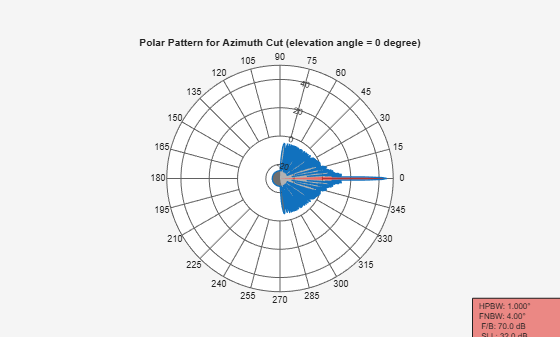Associate the array with the radiator and collector.

radiator = phased.Radiator(... 'Sensor',antenna,'Polarization','Dual',... 'OperatingFrequency',fc); collector = phased.Collector(... 'Sensor',antenna,'Polarization','Dual',... 'OperatingFrequency',fc); 

### Weather Target

Generally, weather radar data is categorized into three levels. Level-I data is raw time series I/Q data as input to the signal processor in the Radar Data Acquisition unit. Level-II data consists of the radar spectral moments (reflectivity, mean radial velocity, and spectrum width) and polarimetric moments (differential reflectivity, correlation coefficient, and differential phase) output from the signal processor. Level-III data is the output product data of the radar product generator, such as hydrometeor classification, storm total precipitation, and tornadic vortex signature.

In this example, Level-II data from KTLX NEXRAD radar at 20:08:11 UTC on May 20th, 2013 is used. This data comes from an intense tornado that occurred in Moore, Oklahoma and is used to generate mean radar cross section (RCS) of equivalent scattering centers. The data is available via FTP download. It represents a volume scan that includes a series of 360-degree sweeps of the antenna at predetermined elevation angles completed in a specified period of time. In the data file name KTLX20130520_200811_V06, KTLX refers to the radar site name, 20130520_200811 refers to the date and time when the data was collected, and V06 refers to the data format of version 6. In this simulation, the lowest elevation cut (0.5 degree) is extracted from the volume scan data as an example.

Read the Level-II data into the workspace. Store it in the nexrad structure array, which contains all the radar moments as well as an azimuth field that specifies the azimuth angle for each radial data point in the Cartesian coordinate system. For simplicity, load NEXRAD data that was transformed from a compressed file to a MAT-file.

load NEXRAD_data.mat; 

Define an area of interest (AOI) in terms of azimuth and range in Cartesian coordinates.

az1 = 96; % Starting azimuth angle (degree) az2 = 105; % Ending azimuth angle (degree) rg1 = 22750; % Starting range (m) rg2 = 38750; % Ending range (m) % NEXRAD has a blind range of 2000m. blind_rg = 2000; % We define the number of azimuth angles that weather targets occupy in the % two dimensional plane. num_az = 40; % We define the number of range bins that weather targets occupy in each % azimuth radial of the two dimensional plane. num_bin = 150; % Select AOI data and store it in _nexrad_aoi_ structure array, which % contains all the radar moments, as well as starting and ending azimuth % and range indices. And the number of available weather targets in space % is returned as Ns. [nexrad_aoi,Ns] = helperSelectAOI(nexrad,az1,az2,rg1,rg2,blind_rg,range_res,num_az,num_bin); 

Because weather targets are polarimetric and distributed in a plane, they can be represented by specifying scattering matrices at discrete azimuth angles. Weather target reflectivity is defined as the mean backscattering cross section per unit volume. Based on the weather radar equation, weather targets can be considered as a collection of small targets within each resolution volume. The overall reflectivity can be transformed to the mean RCS and regarded as an equivalent scattering center. As a result, each element in the scattering matrix is the square root of RCS in relevant polarization.

% Preallocate target position, velocity, RCS, azimuth, elevation, and radar % scattering matrices. tgtpos = zeros(3, Ns); tgtvel = zeros(3, Ns); RCSH = zeros(1,Ns); RCSV = zeros(1,Ns); azpatangs = [-180 180]; elpatangs = [-90 90]; shhpat = zeros(2,2,Ns); svvpat = zeros(2,2,Ns); shvpat = zeros(2,2,Ns); zz = 0; % NEXRAD beamwidth is about 1 degree. beamwidth = 1.0; for ii = nexrad_aoi.rlow:nexrad_aoi.rup theta = nexrad.azimuth(ii); for jj = 1:num_bin if isnan(nexrad.ZH(ii,jj))==0 zz = zz+1; rpos = (jj-1)*range_res + blind_rg; tpos = [rpos*cosd(theta);rpos*sind(theta);0]; tgtpos(:,zz) = tpos; RCSH(zz) = helperdBZ2RCS(beamwidth,rpos,lambda,pulse_width,nexrad.ZH(ii,jj),prop_speed); shhpat(:,:,zz) = sqrt(RCSH(zz))*ones(2,2); RCSV(zz) = helperdBZ2RCS(beamwidth,rpos,lambda,pulse_width,nexrad.ZV(ii,jj),prop_speed); svvpat(:,:,zz) = sqrt(RCSV(zz))*ones(2,2); end end end tgtmotion = phased.Platform('InitialPosition',tgtpos,'Velocity',tgtvel); target = phased.BackscatterRadarTarget('EnablePolarization',true,... 'Model','Nonfluctuating','AzimuthAngles',azpatangs,... 'ElevationAngles',elpatangs,'ShhPattern',shhpat,'ShvPattern',shvpat,... 'SvvPattern',svvpat,'OperatingFrequency',fc); 

Generate a radar data cube using the defined radar system parameters. Within each resolution volume, include the appropriate correlation to ensure the resulting I/Q data presents proper weather signal statistical properties.

rxh_aoi = complex(zeros(nexrad_aoi.rgnum,nexrad_aoi.aznum)); rxv_aoi = complex(zeros(nexrad_aoi.rgnum,nexrad_aoi.aznum)); % The number of realization sequences realiznum = 1000; % The number of unusable range bins due to NEXRAD blind range i0 = blind_rg/range_res; % Rotate sensor platform to simulate NEXRAD scanning in azimuth for kk = 1:nexrad_aoi.aznum axes = rotz(nexrad.azimuth(kk+nexrad_aoi.r1-1)); % Update sensor and target positions [sensorpos,sensorvel] = sensormotion(1/prf); [tgtpos,tgtvel] = tgtmotion(1/prf); % Calculate the target angles as seen by the sensor [tgtrng,tgtang] = rangeangle(tgtpos,sensorpos,axes); % Form transmit beam for this scan angle and simulate propagation pulse = waveform(); [txsig,txstatus] = transmitter(pulse); % Adopt simultaneous transmission and reception mode as NEXRAD txsig = radiator(txsig,txsig,tgtang,axes); txsig = channel(txsig,sensorpos,tgtpos,sensorvel,tgtvel); % Reflect pulse off of targets ang_az = tgtang(1:2:end); ang_az = ang_az+(-1).^(double(ang_az>0))*180; tgtsig = target(txsig,[ang_az;zeros(size(ang_az))],axes); % Collect the target returns received at the sensor [rxsig_h,rxsig_v] = collector(tgtsig,tgtang,axes); rxh = receiver(rxsig_h,~(txstatus>0)); rxv = receiver(rxsig_v,~(txstatus>0)); % Matched filtering [rxh, mfgainh] = matchedfilter(rxh); [rxv, mfgainv] = matchedfilter(rxv); rxh = [rxh(matchingdelay+1:end);zeros(matchingdelay,1)]; rxv = [rxv(matchingdelay+1:end);zeros(matchingdelay,1)]; % Decimation rxh = rxh(1:2:end); rxv = rxv(1:2:end); % Discard blind range data and select AOI data rxh_aoi(:,kk) = rxh(nexrad_aoi.b1+i0:nexrad_aoi.b2+i0); rxv_aoi(:,kk) = rxv(nexrad_aoi.b1+i0:nexrad_aoi.b2+i0); end clear txsig clear tgtsig 

Using pulse pair processing, calculate all the radar moments from estimates of correlations, including reflectivity, mean radial velocity, spectrum width, differential reflectivity, correlation coefficient, and differential phase.

moment = helperWeatherMoment(rxh_aoi,rxv_aoi,nexrad_aoi,pulnum,realiznum,prt,lambda); 

### Simulation Result

Compare the simulation result with the NEXRAD ground truth. Evaluate the simulated data quality using error statistics, a sector image, a range profile, and a scatter plot. Error statistics are expressed as the bias and standard deviation of the estimated radar moments compared to the NEXRAD Level-II data (truth fields).

Define the azimuth and range for plotting.

azimuth = nexrad.azimuth(nexrad_aoi.r1:nexrad_aoi.r2); range = (nexrad_aoi.b1-1:nexrad_aoi.b2-1)*250 + 2000; 

Reflectivity

Reflectivity,, is the zeroth moment of the Doppler spectrum and is related to liquid water content or precipitation rate in the resolution volume. Because values ofthat are commonly encountered in weather observations span many orders of magnitude, radar meteorologists use a logarithmic scale given byas dBZ, whereis in units of mm^6/m^3.

[Z_bias,Z_std] = helperDataQuality(nexrad_aoi,moment,range,azimuth,'Z');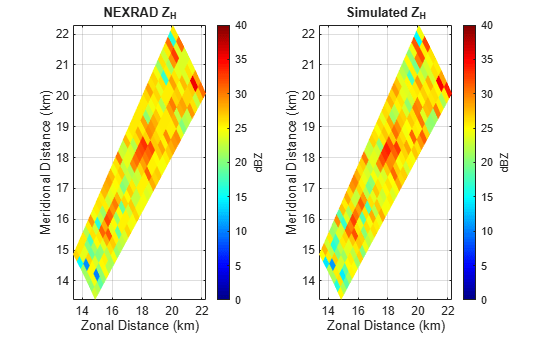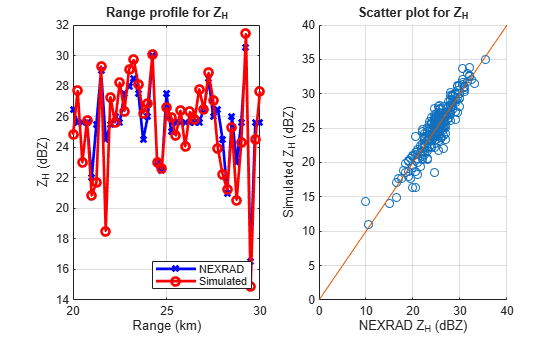Radial velocity,, is the first moment of the power-normalized spectra, which reflects the air motion toward or away from the radar.

[Vr_bias,Vr_std] = helperDataQuality(nexrad_aoi,moment,range,azimuth,'Vr');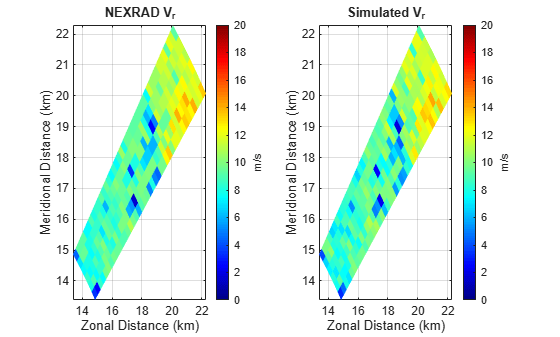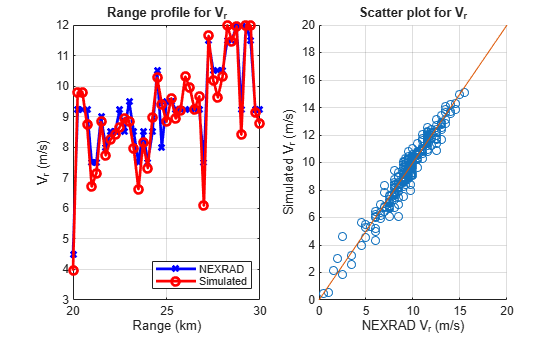Spectrum Width

Spectrum width,, is the square root of the second moment of the normalized spectrum. The spectrum width is a measure of the velocity dispersion, that is, shear or turbulence within the resolution volume.

[sigmav_bias,sigmav_std] = helperDataQuality(nexrad_aoi,moment,range,azimuth,'sigmav');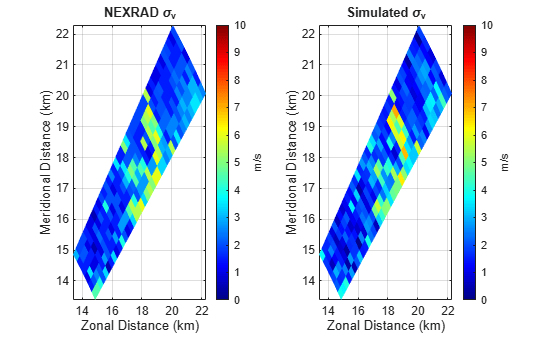Differential Reflectivity

Differential reflectivity,, is estimated from the ratio of the power estimates for the horizontal and vertical polarization signals. The differential reflectivity is useful in hydrometeor classification.

[ZDR_bias,ZDR_std] = helperDataQuality(nexrad_aoi,moment,range,azimuth,'ZDR');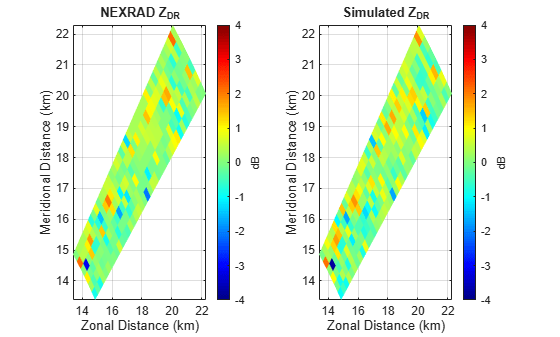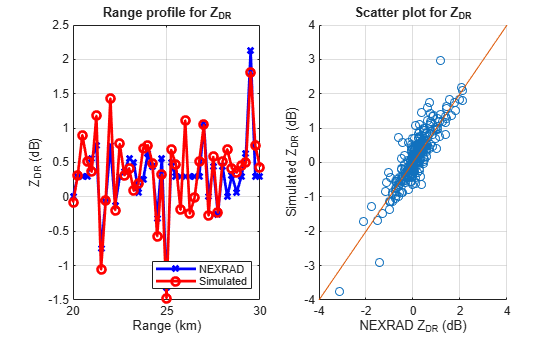Correlation Coefficient

The correlation coefficient,, represents the consistency of the horizontal and vertical returned power and phase for each pulse. The correlation coefficient plays an important role in determining system performance and classifying radar echo types.

[Rhohv_bias,Rhohv_std] = helperDataQuality(nexrad_aoi,moment,range,azimuth,'Rhohv');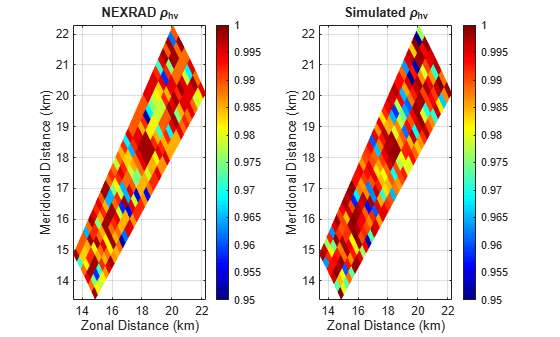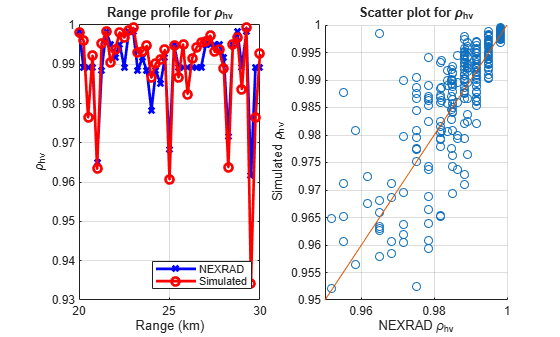Differential Phase

The differential phase,, is the difference in the phase delay of the returned pulse from the horizontal and vertical polarizations. The differential phase provides information on the nature of the scatterers that are being sampled.

[Phidp_bias,Phidp_std] = helperDataQuality(nexrad_aoi,moment,range,azimuth,'Phidp');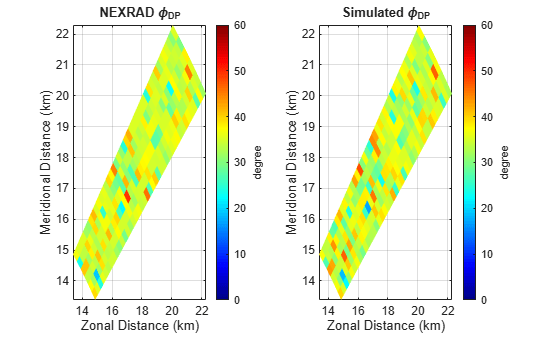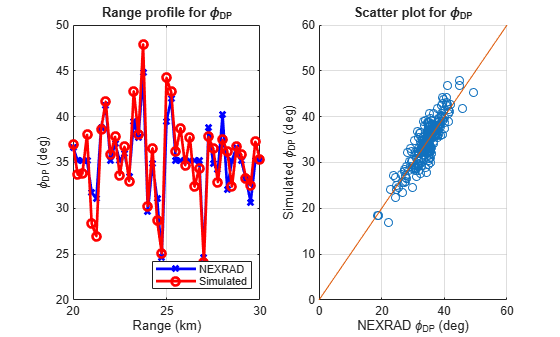Error Statistics

Figures in previous section provide a visual qualitative measure of the simulation quality. This section of the example shows the quantitative comparison of the estimates with NEXRAD specifications as error statistics.

MomentName = {'Z';'Vr';'sigmav';'ZDR';'Rhohv';'Phidp'}; STDEV = [round(Z_std,2);round(Vr_std,2);round(sigmav_std,2);round(ZDR_std,2);round(Rhohv_std,3);round(Phidp_std,2)]; Specs = [1;1;1;0.2;0.01;2]; Unit = {'dB';'m/s';'m/s';'dB';'';'degree'}; T = table(MomentName,STDEV,Specs,Unit); disp(T); 
 MomentName STDEV Specs Unit __________ _____ _____ __________ {'Z' } 0.5 1 {'dB' } {'Vr' } 0.01 1 {'m/s' } {'sigmav'} 0.14 1 {'m/s' } {'ZDR' } 0.06 0.2 {'dB' } {'Rhohv' } 0.006 0.01 {0x0 char} {'Phidp' } 0.16 2 {'degree'} 

By comparison, all the radar moment estimation meets NEXRAD specifications, which indicates good data quality.

### Summary

This example showed how to simulate the polarimetric Doppler radar return from an area of distributed weather targets. Visual comparison and error statistics showed the estimated radar moments met the NEXRAD ground truth specifications. With this example, you can further explore the simulated time series data in other applications such as waveform design, system performance study, and data quality evaluation for weather radar.

### References

 Doviak, R and D. Zrnic. Doppler Radar and Weather Observations, 2nd Ed. New York: Dover, 2006.

 Zhang, G. Weather Radar Polarimetry. Boca Raton: CRC Press, 2016.

 Li, Z, S. Perera, Y. Zhang, G. Zhang, and R. Doviak. "Time-Domain System Modeling and Applications for Multi-Function Array Radar Weather Measurements." 2018 IEEE Radar Conference (RadarConf18), Oklahoma city, OK, 2018, pp. 1049-1054.